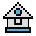Home

# HEX\$ Function

#### Purpose:

To return a string which represents the hexadecimal value of the numeric argument.

#### Syntax:

`v\$=HEX\$(x)`

HEX\$ converts decimal values within the range of -32768 to +65535 into a hexadecimal string expression within the range of 0 to FFFF.

Hexadecimal numbers are numbers to the base 16, rather than base 10 (decimal numbers). Appendix C and Appendix G in the GW-BASIC User's Guide contain more information on hexadecimals and their equivalents.

x is rounded to an integer before HEX\$(x) is evaluated. See the OCT\$ function for octal conversions.

If x is negative, 2's (binary) complement form is used.

#### Examples:

```10 CLS: INPUT "INPUT DECIMAL NUMBER";X
20 A\$=HEX\$(X)
30 PRINT X "DECIMAL IS "A\$" HEXADECIMAL"
RUN
ĀINPUT DECIMAL NUMBER? 32